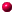"Inside  every small problem is a large problem struggling to get out."

RulesForumContributors[For contributors only]

## Thread and Full Text View

Ask a question about: Zero Sum Games
Respond to the question: solving a zero sum game?

 05/25/2007 02:22 PM by Poonam; Magic square game
Can any body tell me the solution of magic square game.Intutively i think the solution will be like this :
Each player will give probability equal to 1/sum to each strategy.where sum is the sum of all entries in each row.
Am I correct? [Manage messages]

 04/06/2006 03:42 AM by Ali; solving a zero sum game
Is there any other methods of solving a zero sum game(without using linear programming)? [View full text and thread]